# Design of FRP Axial Strengthening of RCC Columns -ACI 440.2R-08Reading time: 1 minute

FRP axial strengthening systems are used to improve or enhance the capacity of reinforced concrete columns. It can be used for both circular and rectangular shaped columns but it is more effective in the former shape. In this article the design of FRP axial strengthening system for columns is discussed.Fig.1: Applying Continuous FRP Systems for Axial Strengthening of RCC Columns

## Advantages of FRP Axial Strengthening Systems for Columns

• Increases the ultimate load carrying capacity of reinforced concrete member
• Improves shear capacity of reinforced concrete element
• Reinforcement bar lap splice capacity of the member is improved due to FRP axial strengthening system application
• The ductility of reinforced concrete column is improved considerably.

## Design of Axial FRP Strengthening Systemsfor Columns

FRP axial strengthening is usually conducted by providing fiber reinforced polymer (FRP) around reinforced concrete columns. This strengthening technique is specifically influential when the column is circular. However, if the reinforced concrete column is rectangular and the ratio of depth to width of column is larger than 2 or the smallest side of the column is greater than 900mm, then ACI 440.2R-08 is not applied for this strengthening method. Figure-2 illustrates the confined area in different shapes of concrete columns.Fig.2: Confined Area in Circular, Square and Rectangular Concrete Columns

The ineffectiveness of rectangular or square column confinement might be attributed to the non-uniform stress distribution and stress concentration at the corner of the section. This may lead to premature failure of strengthened element. It is essential to wrap reinforced concrete column completely with FRPs in order confine and improve the element effectively. Unlike the flexural and shear strengthening of reinforced concrete beams, the FRPs which surround the column activated only if the member is enlarged laterally and exert stresses on the FRPs. This means that, beam strengthening is an active system whereas column strengthening is a passive system. The FRP system which wrapped around the column creates circumferentially uniform confine pressure that acts against the radial compression enlargement. Figure-3 illustrates how FRP systems create a pressure against the compression expansion of the concrete column.Fig.3: Forces Acted in a Confined Concrete ColumnFig.4: Reinforced Concrete Column Confined with Continuous FRPs

### Strengthening of RCC Column Under Pure Compression

The compression strength of reinforced concrete column can be increased through confinement of the column. The confinement is achieved by directing FRP systems around the column transverse to longitudinal direction of the column. It should be said that, any FRP system that applied to in other directions should be ignored. The nominal capacity of short, non-prestressed, normal weight reinforced concrete column with tie and spiral reinforcement can be calculated by the following two equations which is provided by ACI 318-11: For tie: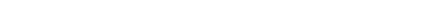For spiral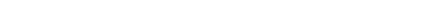The confined compressive strength is estimated using formula provided by ACI 440.2R-08: If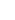is larger than zero but smaller than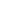, the following formula is applied: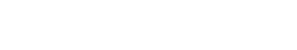Whenis larger thanbut smaller than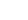, then the following expression is used: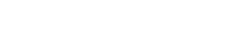Where: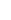: Strength reduction factor which 0.75 for spiral and 0.65 for tie fc': Concrete strength Ag: Gross concrete area Ast: Longitudinal reinforcement area fy: yield strength of longitudinal reinforcement fc: confined compressive strength Ec: Concrete modulus of elasticity: Concrete strain: Ultimate axial compressive strain of confined concrete and it can be computed by applying equation 10 and its value should not exceed 0.01. E2 andare slope of linear portion of stress strain model for FRP confined concrete and transition strain in stress strain curve of FRP confined concrete respectively and both may be estimated by the following equations:The maximum confined concrete compressive strength (fcc') in equation-5 is calculated as follows: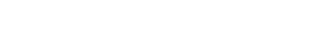Where: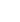: Strength reduction factor taken as 0.95 ka: Efficiency factor for FRP reinforcement in determination of maximum confined compressive strength and it depend on geometry of cross section as shown in figure 2. It can be taken as 1 for circular cross section as per the ACI 440.2R-08 recommendation. fl: Maximum confined pressure because of FRP system and it is computed as: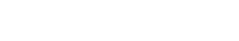Where: Ef: Modulus of elasticity of FRP reinforcement n: Number of plies of FRP reinforcement tf: Nominal thickness of one ply of FRP reinforcement D: diameter of circular cross section compression member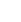: Effective strain level in FRP reinforcement achieved at failure and can be evaluated using the following expression: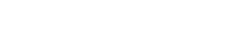Where: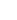: is the FRP strain efficiency factor takes FRP system premature failure into consideration and it is usually taken as 0.58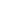: design rupture strain of FRP reinforcement The maximum axial compressive strain of confined concrete can be calculated by the following equations: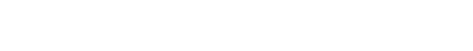Where:: Maximum strain of unconfined concrete kb: Efficiency factor for FRP reinforcement and it can be considered as 1 for circular cross section based on recommendations of ACI 440.2R-08 fl / fc': Confinement ratio and a minimum of 0.08 should be considered as per ACI 440.2R-08 recommendation. If non-circular cross section is strengthened, the equivalent diameter should be used in equation 8 as illustrated in Figure-5:Fig.5: Equivalent Circular Cross Section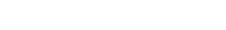And both efficiency factor (ka) in equation-7 and (kb) are based on the cross-sectional area of effectively confined concrete (Ae) and (h?b) ratio as can be observed from the following equations:Where: rc: Radius of the corner of the section as it can be seen from figure 5 pg: Longitudinal steel reinforcement ratio

### Column Subjected to Combined Axial Compression and Bending

Reinforced concrete column that is subjected to both axial compression and bending can be strengthened by axial FRP strengthening systems. If the eccentricity is smaller than 0.1h then equation-1 and equation-2 can be employed to anticipate the confinement effect on the strength improvement. However, when the eccentricity surpasses 0.1h then the two previous equations is employed to estimate the concrete material properties of the cross-section element under compression. This will be used to construct interaction (P-M) diagram, Figure-6, for the concrete element that has been confined by FRP systems. Moreover, there are several restrictions which should be considered when the member is subjected to axial compression and bending. The first condition which should be considered is that, FRP effective strain must be greater than 0.004. Moreover, if maximum applied bending moment and axial force located below the line that connect the balanced point in the interaction diagram for unconfined member and the origin, then strength improvement should not be considered.Fig.6: Interaction Diagram

Read More: Strengthening of RCC Beams in Shear using Externally Bonded FRP Plates or Strips Strengthening of Foundations